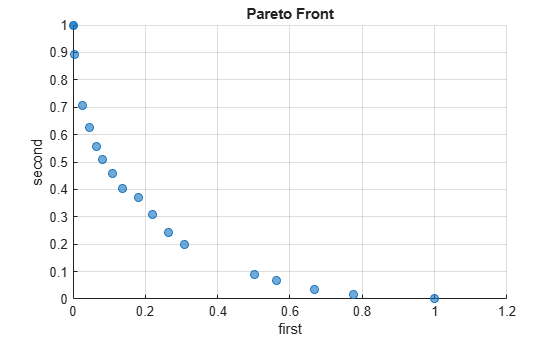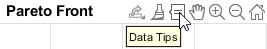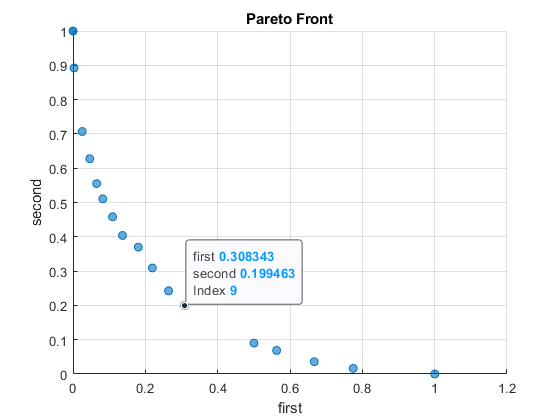# optimproblem

Create optimization problem

## Syntax

``prob = optimproblem``
``prob = optimproblem(Name,Value)``

## Description

Use `optimproblem` to create an optimization problem.

Tip

For the full workflow, see Problem-Based Optimization Workflow.

example

````prob = optimproblem` creates an optimization problem with default properties.```

example

````prob = optimproblem(Name,Value)` uses additional options specified by one or more `Name,Value` pair arguments. For example, to specify a maximization problem instead of a minimization problem, use ```prob = optimproblem('ObjectiveSense','maximize')```. NoteAll names in an optimization problem must be unique. Specifically, all variable names, objective function names, and constraint function names must be different. ```

## Examples

collapse all

Create an optimization problem with default properties.

`prob = optimproblem`
```prob = OptimizationProblem with properties: Description: '' ObjectiveSense: 'minimize' Variables: [0x0 struct] containing 0 OptimizationVariables Objective: [0x0 OptimizationExpression] Constraints: [0x0 struct] containing 0 OptimizationConstraints No problem defined. ```

Create a linear programming problem for maximization. The problem has two positive variables and three linear inequality constraints.

`prob = optimproblem('ObjectiveSense','max');`

Create positive variables. Include an objective function in the problem.

```x = optimvar('x',2,1,'LowerBound',0); prob.Objective = x(1) + 2*x(2);```

Create linear inequality constraints in the problem.

```cons1 = x(1) + 5*x(2) <= 100; cons2 = x(1) + x(2) <= 40; cons3 = 2*x(1) + x(2)/2 <= 60; prob.Constraints.cons1 = cons1; prob.Constraints.cons2 = cons2; prob.Constraints.cons3 = cons3;```

Review the problem.

`show(prob)`
``` OptimizationProblem : Solve for: x maximize : x(1) + 2*x(2) subject to cons1: x(1) + 5*x(2) <= 100 subject to cons2: x(1) + x(2) <= 40 subject to cons3: 2*x(1) + 0.5*x(2) <= 60 variable bounds: 0 <= x(1) 0 <= x(2) ```

Solve the problem.

`sol = solve(prob);`
```Solving problem using linprog. Optimal solution found. ```
`sol.x`
```ans = 2×1 25.0000 15.0000 ```

Create a problem with two objective functions of a 2-D variable `x`. Create the objective functions as expressions in `x`, and place them in the objective as structures.

```x = optimvar("x",2,LowerBound=-2,UpperBound=2); prob = optimproblem; prob.Objective.first = norm(x)^2; prob.Objective.second = norm(x - [1;0])^2;```

Solve the problem.

```rng default % For reproducibility sol = solve(prob);```
```Solving problem using gamultiobj. Optimization terminated: average change in the spread of Pareto solutions less than options.FunctionTolerance. ```

Plot the solution.

`paretoplot(sol)`Examine one point on the Pareto front. To do so, click the figure and click the Data Tips tool:Then click a point on the Pareto front.The index of the pictured point is 9. You can find the `x` value associated with this point as the solution with index 9.

`sol(9).x`
```ans = 2×1 0.5544 -0.0306 ```

## Input Arguments

collapse all

### Name-Value Arguments

Specify optional pairs of arguments as `Name1=Value1,...,NameN=ValueN`, where `Name` is the argument name and `Value` is the corresponding value. Name-value arguments must appear after other arguments, but the order of the pairs does not matter.

Before R2021a, use commas to separate each name and value, and enclose `Name` in quotes.

Example: To specify a maximization problem, use ```prob = optimproblem('ObjectiveSense','maximize')```.

Problem constraints, specified as an `OptimizationConstraint` array or a structure with `OptimizationConstraint` arrays as fields.

Example: ```prob = optimproblem('Constraints',sum(x,2) == 1)```

Problem label, specified as a string or character vector. The software does not use `Description` for computation. `Description` is an arbitrary label that you can use for any reason. For example, you can share, archive, or present a model or problem, and store descriptive information about the model or problem in `Description`.

Example: `"An iterative approach to the Traveling Salesman problem"`

Data Types: `char` | `string`

Objective function, specified as a scalar `OptimizationExpression` object, an array of `OptimizationExpression` objects, or a structure with scalar `OptimizationExpression` as fields.

• For a scalar (single-objective) problem, specify the objective function as a scalar optimization expression or as a structure with a scalar optimization expression as the value.

• For a multiobjective problem, specify the objective functions as a vector-valued optimization expression, as an array of optimization expressions, or as a structure of optimization expressions. For example, this objective is a structure of optimization expressions in a scalar optimization variable `x`:

```prob = optimproblem; prob.Objective.first = x^2; prob.Objective.second = (x + 1)^2;```

Example: ```prob = optimproblem('Objective',sum(sum(x)))``` for a 2-D variable `x`.

Example: `prob = optimproblem('Objective',(x-a).^2)` where `x` and `a` have size 2-by-1, and `x` is an optimization variable.

Sense of optimization, specified as `'minimize'` or `'maximize'`. You can also specify `'min'` to obtain `'minimize'` or `'max'` to obtain `'maximize'`. The `solve` function minimizes an objective when `ObjectiveSense` is `'minimize'` and maximizes an objective when `ObjectiveSense` is `'maximize'`.

`ObjectiveSense` can be a structure with values `'minimize'`, `'min'`, `'maximize'`, or `'max'`. You can use this form when the problem objective is a structure. The `Objective` and `ObjectiveSense` structures should have the same field names, so the `ObjectiveSense` applies to the corresponding `Objective`. For example,

```x = optimvar('x',2,"UpperBound",2,"LowerBound",-2); prob = optimproblem; prob.Objective.first = norm(x)^2; prob.Objective.second = -norm(x - [1;0])^2; prob.ObjectiveSense.first = "min"; prob.ObjectiveSense.second = "max";```

If `Objective` is a structure, you can specify `ObjectiveSense` as a name such as `'max'`. In this case, all objectives have the same `ObjectiveSense`.

Example: ```prob = optimproblem('ObjectiveSense','max')```

Data Types: `char` | `string`

## Output Arguments

collapse all

Optimization problem, returned as an `OptimizationProblem` object. Typically, to complete the problem description, you specify an objective function and constraints. However, you can have a feasibility problem, which has no objective function, or you can have a problem with no constraints. Solve a complete problem by calling `solve`.

Warning

The problem-based approach does not support complex values in an objective function, nonlinear equalities, or nonlinear inequalities. If a function calculation has a complex value, even as an intermediate value, the final result can be incorrect.

## Version History

Introduced in R2017b